Test: Geotechnical Engineering- 2

# Test: Geotechnical Engineering- 2 - Civil Engineering (CE)

Test Description

## 20 Questions MCQ Test GATE Civil Engineering (CE) 2023 Mock Test Series - Test: Geotechnical Engineering- 2

Test: Geotechnical Engineering- 2 for Civil Engineering (CE) 2023 is part of GATE Civil Engineering (CE) 2023 Mock Test Series preparation. The Test: Geotechnical Engineering- 2 questions and answers have been prepared according to the Civil Engineering (CE) exam syllabus.The Test: Geotechnical Engineering- 2 MCQs are made for Civil Engineering (CE) 2023 Exam. Find important definitions, questions, notes, meanings, examples, exercises, MCQs and online tests for Test: Geotechnical Engineering- 2 below.
Solutions of Test: Geotechnical Engineering- 2 questions in English are available as part of our GATE Civil Engineering (CE) 2023 Mock Test Series for Civil Engineering (CE) & Test: Geotechnical Engineering- 2 solutions in Hindi for GATE Civil Engineering (CE) 2023 Mock Test Series course. Download more important topics, notes, lectures and mock test series for Civil Engineering (CE) Exam by signing up for free. Attempt Test: Geotechnical Engineering- 2 | 20 questions in 60 minutes | Mock test for Civil Engineering (CE) preparation | Free important questions MCQ to study GATE Civil Engineering (CE) 2023 Mock Test Series for Civil Engineering (CE) Exam | Download free PDF with solutions
 1 Crore+ students have signed up on EduRev. Have you?
*Answer can only contain numeric values
Test: Geotechnical Engineering- 2 - Question 1

### Given soil sample: Degree of saturation = 90% Specific gravity of soil grains = 2.70 Void ratio = 0.30 The water content of the sample is ________ %.

Detailed Solution for Test: Geotechnical Engineering- 2 - Question 1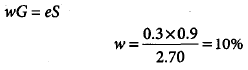*Answer can only contain numeric values
Test: Geotechnical Engineering- 2 - Question 2

### The approximate ratio of the permeability of two clean soils having D10 = 0.6 mm and D10 = 0.3 mm is __________.

Detailed Solution for Test: Geotechnical Engineering- 2 - Question 2

We know that
K = CD102
where C is shape co-efficient.
Therefore, approximate ratio will be = (0.62)/(0.32) = 4

Test: Geotechnical Engineering- 2 - Question 3

### Two soil samples A and B have porosities nA = 40% and nB = 60% respectively. What is the ratio of void ratio eA : eB ?

Detailed Solution for Test: Geotechnical Engineering- 2 - Question 3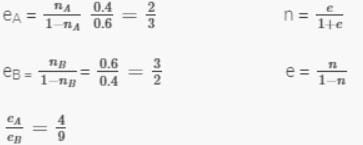Test: Geotechnical Engineering- 2 - Question 4

Which one of the following equation correctly gives the relationship between the specific gravity of soil grains (G) and the hydraulic gradient (i) of initiate “quick” condition in sand having a void ratio of 0.5?

Test: Geotechnical Engineering- 2 - Question 5

If the time required for 60% consolidation of a remoulded soil sample of clay with simple drainage is t, then what is the time required to consolidation the same sample of clay with the same degree of consolidation but with double drainage?

Detailed Solution for Test: Geotechnical Engineering- 2 - Question 5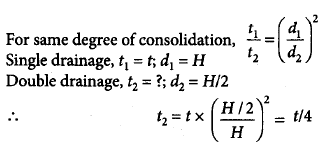Test: Geotechnical Engineering- 2 - Question 6

If s is the shear strength, c and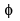are shear strength parameters, and σn is the normal stress at failure, then Coulomb’s equation for shear strength of the soil can be represented by

Detailed Solution for Test: Geotechnical Engineering- 2 - Question 6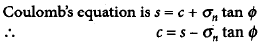Test: Geotechnical Engineering- 2 - Question 7

A wet soil mass is having one-sixth of its volume as air and one-third of its volume as water. The void ratio of the soil is

Detailed Solution for Test: Geotechnical Engineering- 2 - Question 7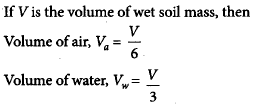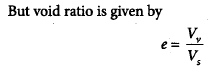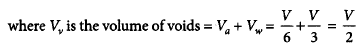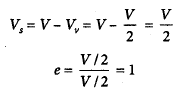Test: Geotechnical Engineering- 2 - Question 8

A soil has liquid limit of 60% plastic limit of 35% and shrinkage limit of 20% and it has a natural moisture content of 50%. The liquidity index of soil is

Detailed Solution for Test: Geotechnical Engineering- 2 - Question 8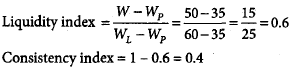Test: Geotechnical Engineering- 2 - Question 9

Match List-I with List-II and select the correct answer using the codes given below: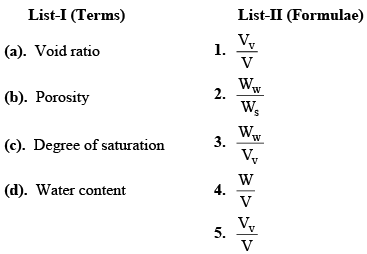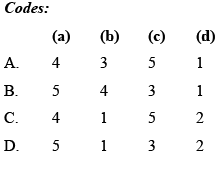Test: Geotechnical Engineering- 2 - Question 10

Negative skin friction on a pile under vertical compressive load acts

Test: Geotechnical Engineering- 2 - Question 11

Two footings, one circular and the other square, are founded on the surface of a purely cohensionless soil. The diameter of the circular footing is same as that of the side of the square footing. The ratio of their ultimate bearing capacities is

Detailed Solution for Test: Geotechnical Engineering- 2 - Question 11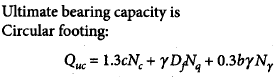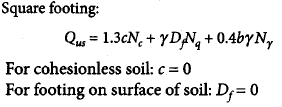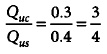Test: Geotechnical Engineering- 2 - Question 12

The liquid limit and plastic limit of sample are 65% and 29% respectively. The percentage of the soil fraction with grain size finer than 0.002 mm is 24. The activity ratio of the soil sample is

Detailed Solution for Test: Geotechnical Engineering- 2 - Question 12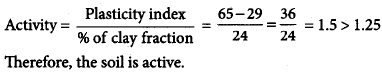Test: Geotechnical Engineering- 2 - Question 13

A concentrated load of 50t act vertically at a point on the soil surface. If Boussinesq’s equation is applied for computation of stress, then the ratio of vertical stresses at depths of 3 m and 5 m, respective, vertically below the point of application of load will be

Detailed Solution for Test: Geotechnical Engineering- 2 - Question 13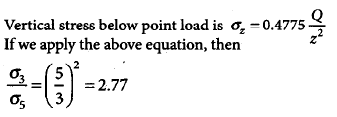Test: Geotechnical Engineering- 2 - Question 14

In a cohension less soil deposit having a unit weight of 1.5 t/m3 and an angle of internal friction of 30°, the active and passive lateral earth pressure intensities (in t/m2) at a depth of 10 m will, respectively, be

Detailed Solution for Test: Geotechnical Engineering- 2 - Question 14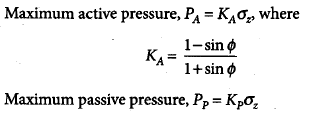Test: Geotechnical Engineering- 2 - Question 15

A raft of 6 m × 9 m is found at a depth of 3 m in a cohesive soil having c = 120 kN/m3. The ultimate net bearing capacity of the soil using the Terzaghi’s theory will be nearly

Detailed Solution for Test: Geotechnical Engineering- 2 - Question 15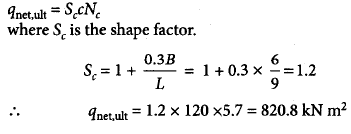Test: Geotechnical Engineering- 2 - Question 16

A stratus of 3.5m thick fine sand has a void ratio of 0.7 and G of 2.7. For a quick sand condition to develop in this stratum, the water flowing in upward direction would require a head of

Detailed Solution for Test: Geotechnical Engineering- 2 - Question 16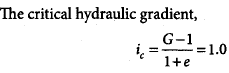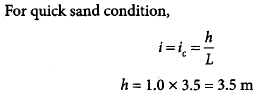*Answer can only contain numeric values
Test: Geotechnical Engineering- 2 - Question 17

The settlement analysis for a clay layer draining from top and bottom shows a settlement of 2.5 cm in 4 years and an ultimate settlement of 10 cm. However, detailed subsurface investigation reveals that there is non drainage at the bottom. The ultimate settlement in this condition will be ______ cm.

Detailed Solution for Test: Geotechnical Engineering- 2 - Question 17

The ultimate settlement is neither dependent on time nor dependent on drainage conditions. Thus, the ultimate settlement remains same equal to 10 cm.

Test: Geotechnical Engineering- 2 - Question 18

A strip footing having 1.5 m width founded at a depth of 3m below ground level in a clay soil having c = 20 kN/m2. ϕ = 0° and unit weight γ = 20 kN/m3. What is the net ultimate bearing capacity using Skempton’s analysis?

Detailed Solution for Test: Geotechnical Engineering- 2 - Question 18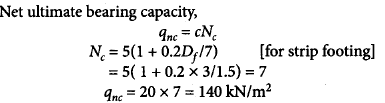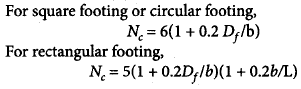Test: Geotechnical Engineering- 2 - Question 19

A retaining wall with a vertical back of height 7.32 m supports a cohesionless soil of unit weight 17.3 kN/m3 and an angle of shearing resistance= 300. Given the surface of the soil is horizontal.

Q.

The coefficient of active earth pressure, using Rankine theory, will be

Detailed Solution for Test: Geotechnical Engineering- 2 - Question 19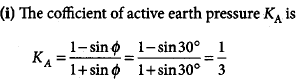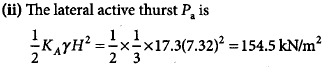Test: Geotechnical Engineering- 2 - Question 20

A retaining wall with a vertical back of height 7.32 m supports a cohesionless soil of unit weight 17.3 kN/m3 and an angle of shearing resistance= 300. Given the surface of the soil is horizontal.

Q.

The active thrust per meter of wall using Rankine theory is

Detailed Solution for Test: Geotechnical Engineering- 2 - Question 20## GATE Civil Engineering (CE) 2023 Mock Test Series

27 docs|296 tests
Information about Test: Geotechnical Engineering- 2 Page
In this test you can find the Exam questions for Test: Geotechnical Engineering- 2 solved & explained in the simplest way possible. Besides giving Questions and answers for Test: Geotechnical Engineering- 2, EduRev gives you an ample number of Online tests for practice

## GATE Civil Engineering (CE) 2023 Mock Test Series

27 docs|296 tests(Scan QR code)